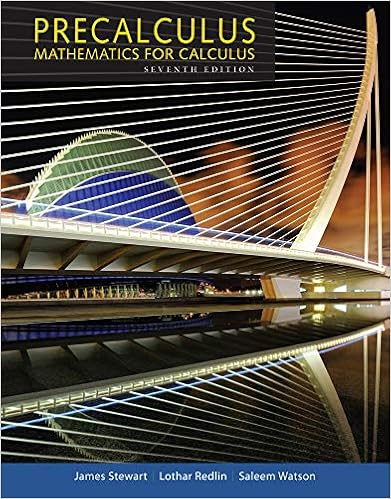# stat331-prac1.pdf - STAT 331 Applied Linear Models Midterm...

• Test Prep
• nitishaagarwal74
• 5
• 100% (1) 1 out of 1 people found this document helpful

This preview shows page 1 - 3 out of 5 pages.

##### We have textbook solutions for you!
The document you are viewing contains questions related to this textbook.The document you are viewing contains questions related to this textbook.
Chapter 1 / Exercise 14
Precalculus: Mathematics for Calculus
Redlin/StewartExpert Verified
STAT 331: Applied Linear ModelsMidterm 1: Practice Problemsversion: 2018-10-03·18:35:59Q1.Consider the simple linear regression modelyiind∼ N(β0+β1xi, σ2),i=1, . . . ,n.a(a)Write down expressionsˆβ0andˆβ1for the MLEs ofβ0andβ1in terms of ¯x, ¯y,Sxyandsxx.(b)Forn=20, find the ratioρ=ˆσ/ˆσ(ML)of the unbiased estimator to the MLE ofσ.(c)Suppose thatn=50 andSxx=180,¯x=4.8,Sxy=353,nXi=1xiyi=3378.Calculate the values of the MLEsˆβ0andˆβ1.Q2.Consider the simple linear regression modelyiind∼ N(β0+β1xi, σ2),i=1, . . . ,n.Suppose thatn=10 andˆβ1=5,ˆβ0=-2,Sxx=16,¯x=0,ˆσ=0.4.Moreover, ifTt(8), we have the following information:Pr(T>-1.86)=.95Pr(|T|>2.75)=.025Pr(T<2.31)=.975a(a)Calculate a two-sided 95% confidence interval forβ1.(b)Calculate an upper 95% prediction bound fory?=β0+β1x?+ε?at the value ofx?=5.1
##### We have textbook solutions for you!
The document you are viewing contains questions related to this textbook.The document you are viewing contains questions related to this textbook.
Chapter 1 / Exercise 14
Precalculus: Mathematics for Calculus
Redlin/StewartExpert Verified
Midterm 1: Practice Problems
•••